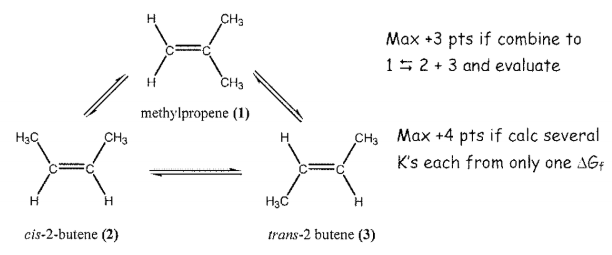# Problem: At 25 °C, 1.0 M methylpropene (1) was added to a reaction vessel. Upon addition of a suitable catalyst, an equilibrium mixture of three isomeric compounds formed. Methylpropene (1), cis-2-butene (2), and trans-2 butene (3) are isomers with formula C4H8, with ΔG°f = +58.07 kJ/mol, +65.86 kJ/mol and +62.97 kJ/mol, respectively. What will be the concentration of each isomer present at 25 °C once equilibrium is established? Please circle your answer(s)Hint: The sum of the equilibrium concentrations of the three species will still total 1.0 M.

###### FREE Expert Solution

We are asked to calculate the concentration of each isomer present at 25 °C once equilibrium is established.

Recall that the equilibrium concentrations are determined by the equilibrium constant (K):

Recall that ΔG˚rxn and K are related to each other:

$\overline{){\mathbf{\Delta G}}{{\mathbf{°}}}_{{\mathbf{rxn}}}{\mathbf{=}}{\mathbf{-}}{\mathbf{RTlnK}}}$

With this, we can derive expressions of K.

We can use the following equation to solve for ΔG˚rxn:

We go through the following steps to solve the problem:

Step 1: Calculate ΔG°rxn for (1) → (2) and (1) → (3)

Step 2: Derive K expressions for (1) → (2) and (1) → (3)

Step 3: Calculate the concentrations of each

82% (104 ratings)###### Problem Details

At 25 °C, 1.0 M methylpropene (1) was added to a reaction vessel. Upon addition of a suitable catalyst, an equilibrium mixture of three isomeric compounds formed. Methylpropene (1), cis-2-butene (2), and trans-2 butene (3) are isomers with formula C4H8, with ΔG°f = +58.07 kJ/mol, +65.86 kJ/mol and +62.97 kJ/mol, respectively. What will be the concentration of each isomer present at 25 °C once equilibrium is established? Please circle your answer(s)

Hint: The sum of the equilibrium concentrations of the three species will still total 1.0 M.